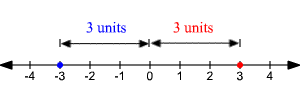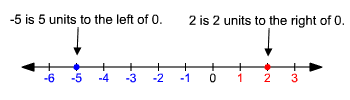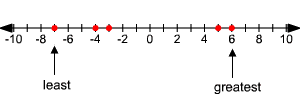# Comparing and Ordering Integers

Integers are the set of positive whole numbers, their opposites, and zero.

Two numbers that are the same distance from $0$ on a number line, but in opposite directions, are opposites.

Example:

Find the opposite of $-3$ .The opposite of $-3$ is $+3$ , or $3$ , because $-3$ and $+3$ are each three units from $0$ , but in opposite directions.

You can compare and order integers by graphing. Numbers increase in value from left to right.

### Comparing Integers

Compare $-5$ and $2$ using $<$ , $>$ , or $=$ .Since $-5$ is to the left of $2$ on the number line , $-5<2$ .

### Ordering Integers

In golf, the person with the lowest score is the winner. Rank the players at the right by ordering their scores from lowest to highest.

 Player Score T.Woods $-7$ V.Singh $-4$ E.Els $+5$ P.Mickelson $-3$ R.Goosen $+6$

Graph the scores on a number line.From lowest to highest scores, the players are T.Woods, V.Singh, P.Mickelson, E.Els, and R.Goosen.